# Chapter 21 Electric Charge 21 2 Electric Charge

• Slides: 18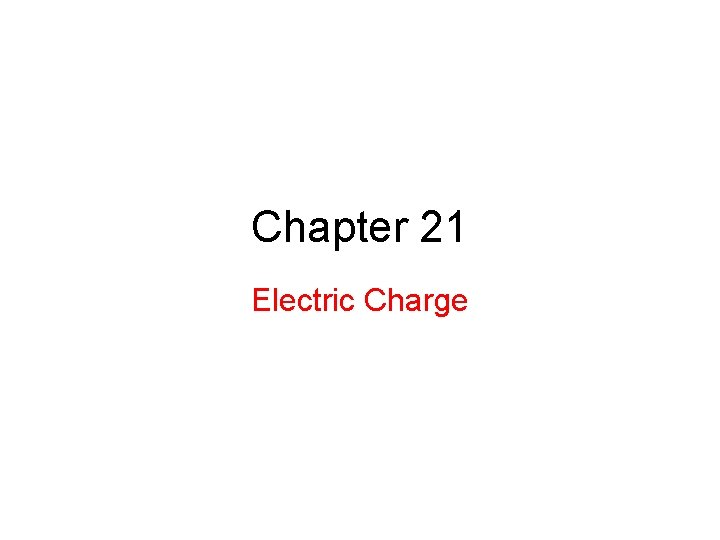Chapter 21 Electric Charge21. 2 Electric Charge: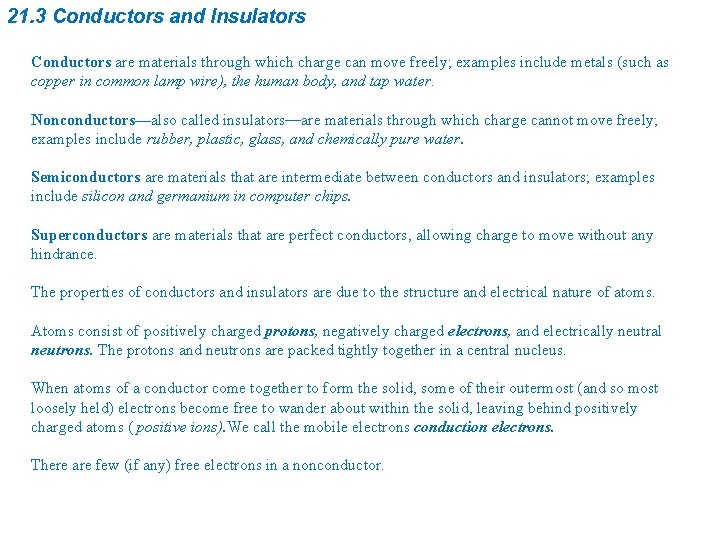21. 3 Conductors and Insulators Conductors are materials through which charge can move freely; examples include metals (such as copper in common lamp wire), the human body, and tap water. Nonconductors—also called insulators—are materials through which charge cannot move freely; examples include rubber, plastic, glass, and chemically pure water. Semiconductors are materials that are intermediate between conductors and insulators; examples include silicon and germanium in computer chips. Superconductors are materials that are perfect conductors, allowing charge to move without any hindrance. The properties of conductors and insulators are due to the structure and electrical nature of atoms. Atoms consist of positively charged protons, negatively charged electrons, and electrically neutral neutrons. The protons and neutrons are packed tightly together in a central nucleus. When atoms of a conductor come together to form the solid, some of their outermost (and so most loosely held) electrons become free to wander about within the solid, leaving behind positively charged atoms ( positive ions). We call the mobile electrons conduction electrons. There are few (if any) free electrons in a nonconductor.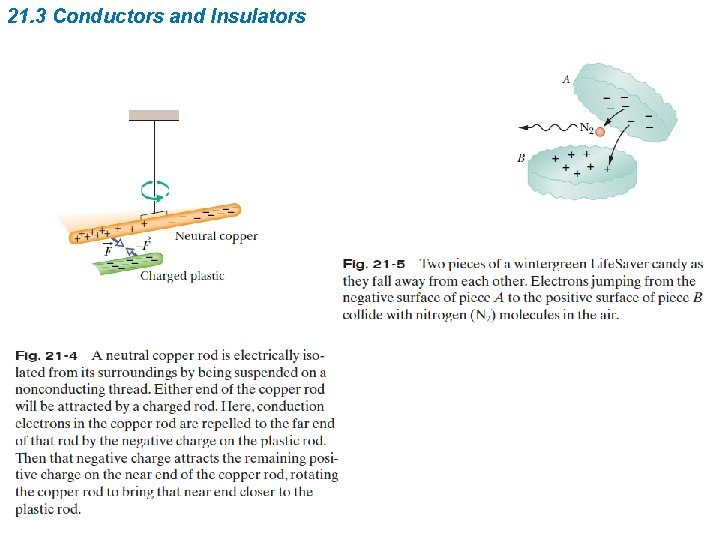21. 3 Conductors and Insulators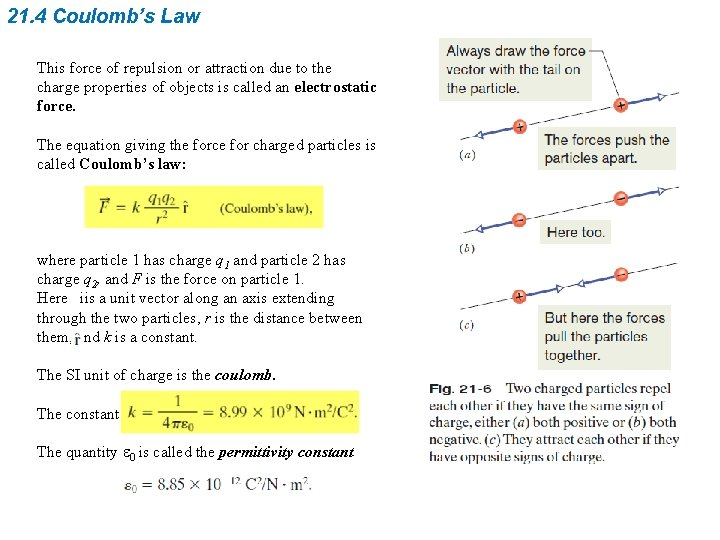21. 4 Coulomb’s Law This force of repulsion or attraction due to the charge properties of objects is called an electrostatic force. The equation giving the force for charged particles is called Coulomb’s law: where particle 1 has charge q 1 and particle 2 has charge q 2, and F is the force on particle 1. Here iis a unit vector along an axis extending through the two particles, r is the distance between them, and k is a constant. The SI unit of charge is the coulomb. The constant The quantity e 0 is called the permittivity constant21. 4 Coulomb’s Law Current is the rate dq/dt at which charge moves past a point or through a region in which i is the current (in amperes) and dq (in coulombs) is the amount of charge moving past a point or through a region in time dt (in seconds). Therefore,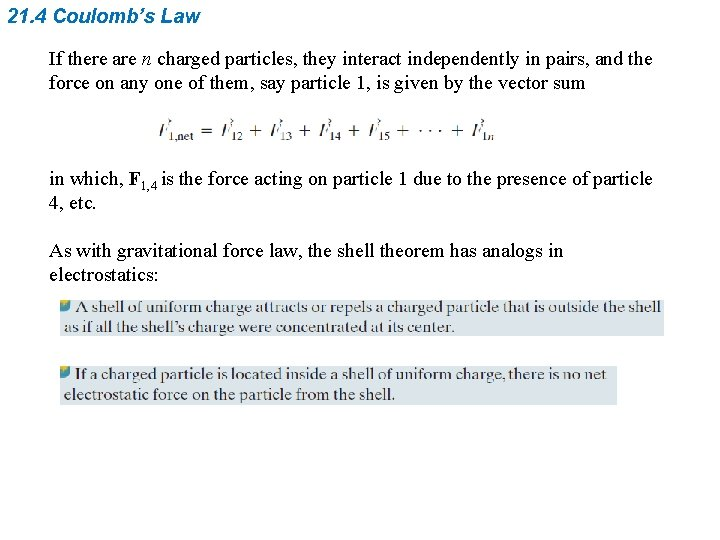21. 4 Coulomb’s Law If there are n charged particles, they interact independently in pairs, and the force on any one of them, say particle 1, is given by the vector sum in which, F 1, 4 is the force acting on particle 1 due to the presence of particle 4, etc. As with gravitational force law, the shell theorem has analogs in electrostatics:Example, The net force due to two other particles: Fig. 21 -8 (a) Two charged particles of charges q 1 and q 2 are fixed in place on an x axis. (b) The free-body diagram for particle 1, showing the electrostatic force on it from particle 2.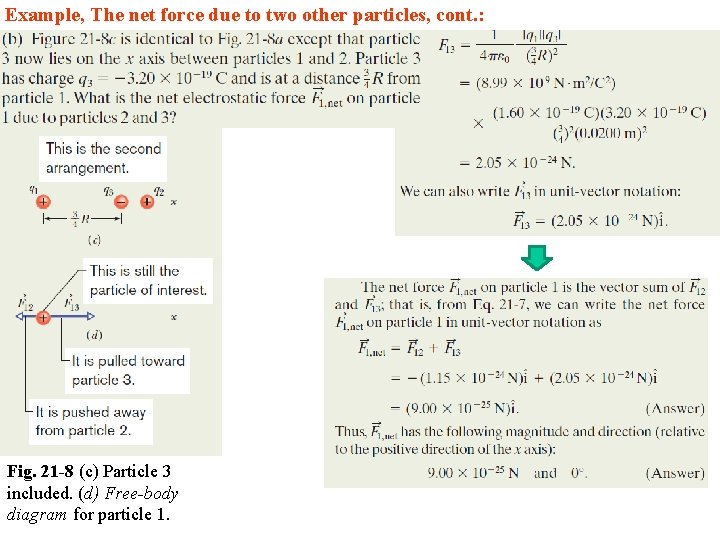Example, The net force due to two other particles, cont. : Fig. 21 -8 (c) Particle 3 included. (d) Free-body diagram for particle 1.Example, The net force due to two other particles, cont. : Fig. 21 -8 (e) Particle 4 included. (f ) Freebody diagram for particle 1.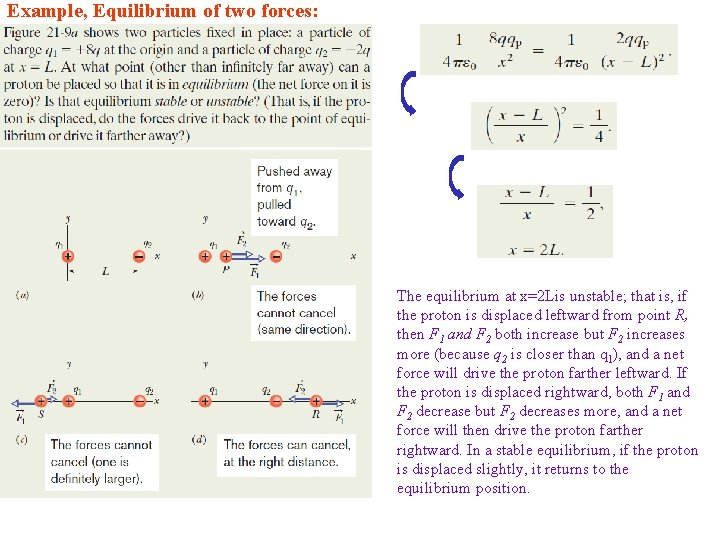Example, Equilibrium of two forces: The equilibrium at x=2 Lis unstable; that is, if the proton is displaced leftward from point R, then F 1 and F 2 both increase but F 2 increases more (because q 2 is closer than q 1), and a net force will drive the proton farther leftward. If the proton is displaced rightward, both F 1 and F 2 decrease but F 2 decreases more, and a net force will then drive the proton farther rightward. In a stable equilibrium, if the proton is displaced slightly, it returns to the equilibrium position.Example, Charge Sharing: (1) Since the spheres are identical, connecting them means that they end up with identical charges (same sign and same amount). (2) The initial sum of the charges (including the signs of the charges) must equal the final sum of the charges. Reasoning: When the spheres are wired together, the (negative) conduction electrons on B move away from one another (along the wire to positively charged A— Fig. 2110 b. ) As B loses negative charge, it becomes positively charged, and as A gains negative charge, it becomes less positively charged. The transfer of charge stops when the charge on B has increased to Q/2 and the charge on A has decreased to Q/2, which occurs when Q/2 has shifted from B to A. The spheres, now positively charged, repel each other. Fig. 21 -10 Two small conducting spheres A and B. (a) To start, sphere A is charged positively. (b) Negative charge is transferred from B to A through a connecting wire. (c) Both spheres are then charged positively.Example, Charge Sharing, cont. : Reasoning: When we provide a conducting path between a charged object and the ground (which is a huge conductor), we neutralize the object. Were sphere A negatively charged, the mutual repulsion between the excess electrons would cause them to move from the sphere to the ground. Fig. 21 -10 (d) Negative charge is transferred through a grounding wire to sphere A. (e) Sphere A is then neutral. However, because sphere A is positively charged, electrons with a total charge of Q/2 move from the ground up onto the sphere (Fig. 21 -10 d), leaving the sphere with a charge of 0 (Fig. 21 -10 e). Thus, there is (again) no electrostatic force between the two spheres.21. 5 Charge is Quantized . Since the days of Benjamin Franklin, our understanding of of the nature of electricity has changed from being a type of ‘continuous fluid’ to a collection of smaller charged particles. The total charge was found to always be a multiple of a certain elementary charge, “e”: The value of this elementary charge is one of the fundamental constants of nature, and it is the magnitude of the charge of both the proton and the electron. The value of “e” is:21. 5 Charge is Quantized Elementary particles either carry no charge, or carry a single elementary charge. When a physical quantity such as charge can have only discrete values, rather than any value, we say the quantity is quantized. It is possible, For example, to find a particle that has no charge at all, or a charge of +10 e, or -6 e, but not a particle with a charge of, say, 3. 57 e.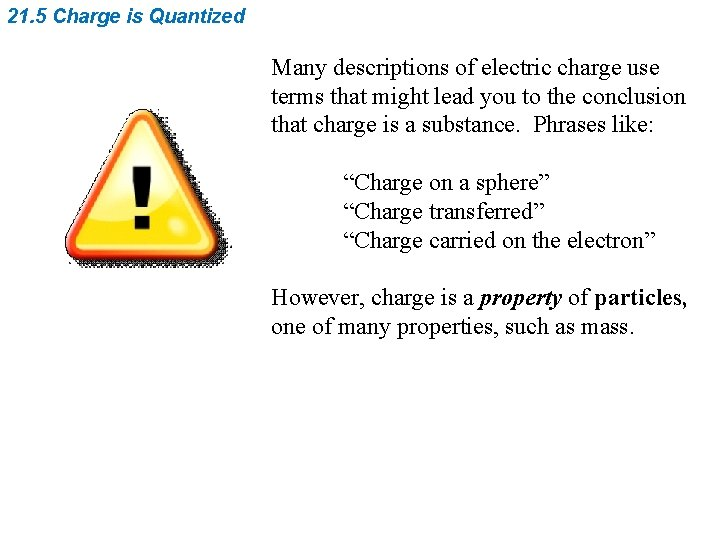21. 5 Charge is Quantized Many descriptions of electric charge use terms that might lead you to the conclusion that charge is a substance. Phrases like: “Charge on a sphere” “Charge transferred” “Charge carried on the electron” However, charge is a property of particles, one of many properties, such as mass.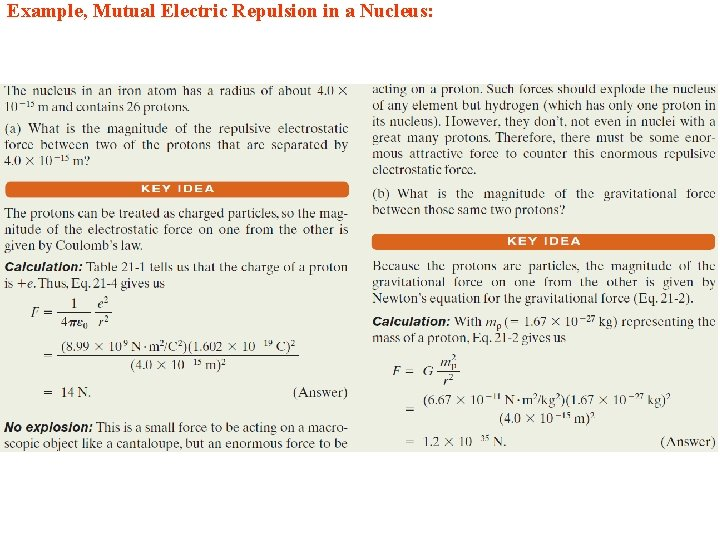Example, Mutual Electric Repulsion in a Nucleus: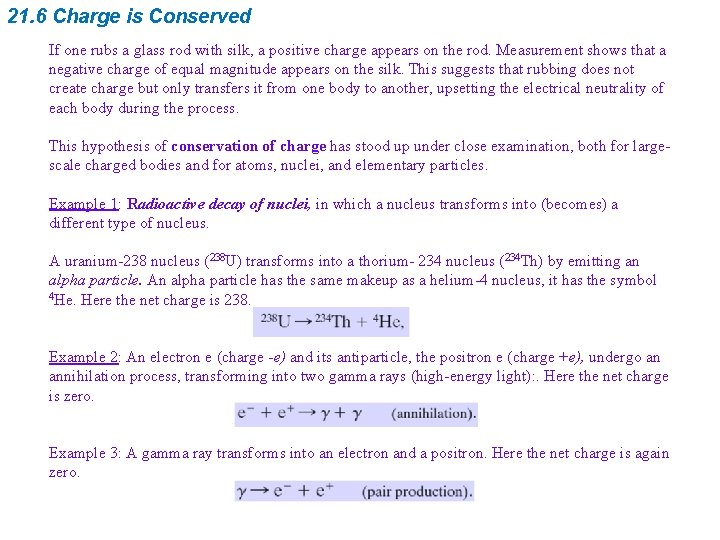21. 6 Charge is Conserved If one rubs a glass rod with silk, a positive charge appears on the rod. Measurement shows that a negative charge of equal magnitude appears on the silk. This suggests that rubbing does not create charge but only transfers it from one body to another, upsetting the electrical neutrality of each body during the process. This hypothesis of conservation of charge has stood up under close examination, both for largescale charged bodies and for atoms, nuclei, and elementary particles. Example 1: Radioactive decay of nuclei, in which a nucleus transforms into (becomes) a different type of nucleus. A uranium-238 nucleus (238 U) transforms into a thorium- 234 nucleus (234 Th) by emitting an alpha particle. An alpha particle has the same makeup as a helium-4 nucleus, it has the symbol 4 He. Here the net charge is 238. Example 2: An electron e (charge -e) and its antiparticle, the positron e (charge +e), undergo an annihilation process, transforming into two gamma rays (high-energy light): . Here the net charge is zero. Example 3: A gamma ray transforms into an electron and a positron. Here the net charge is again zero.